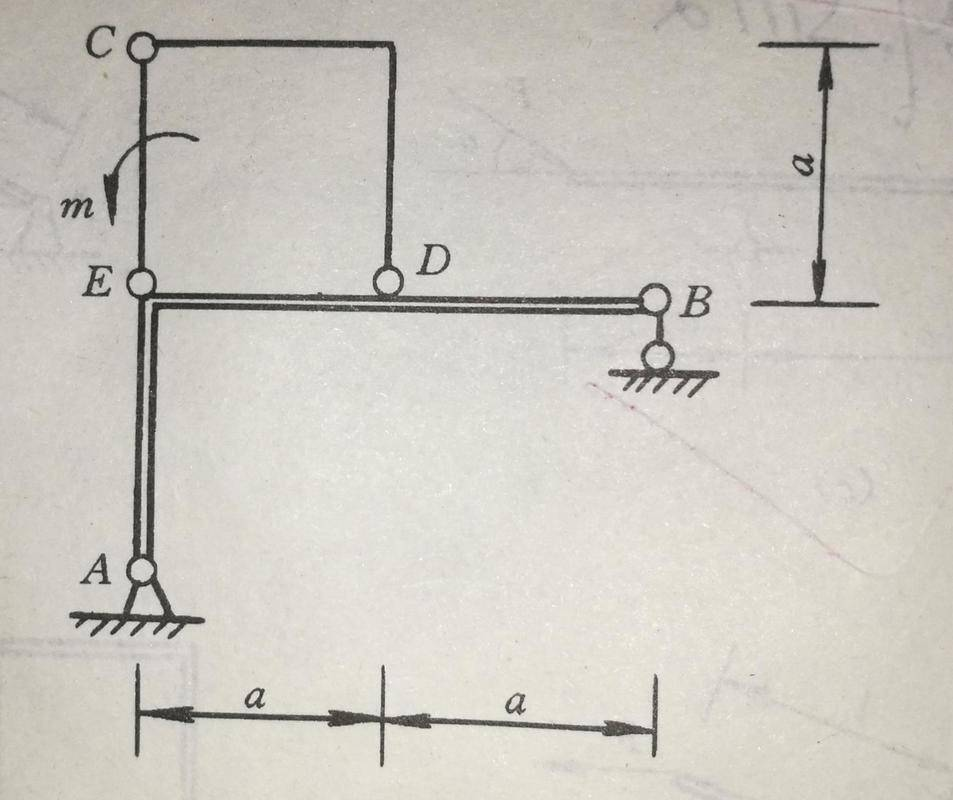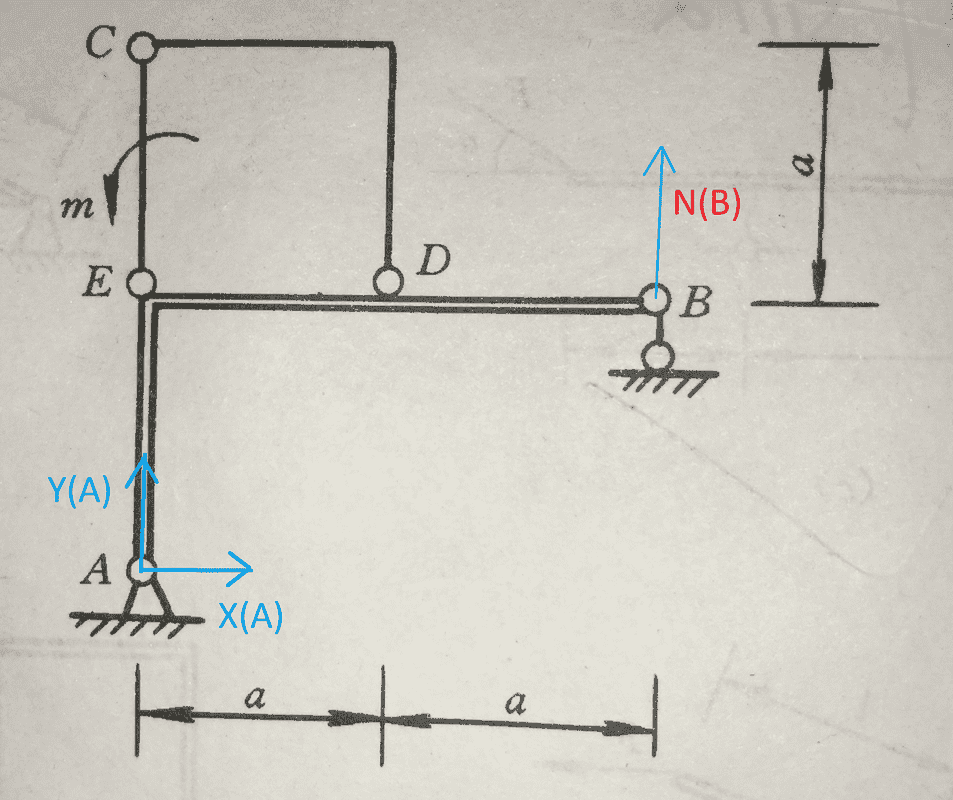# Need help finding the restraining reaction force for a beam

Tags:
1. Mar 14, 2019

### Kile1. The illustrated structure is affected by a known couple, and try to figure out the restraining reaction force of the hinge A and hinge E.We should analyse ECD instead. Since arm CD is a two force members, so N(C) in in the direction where CD connects by these two points. The distance from E to diagonal CD is a/√2. So we have N(C)=√2 m/a. Because N(C)=N(E) ( N(C) and N(E) together form a couple), N(E)=√2 m/a.
Where did I go wrong?

2.3. Mar 14, 2019

### haruspexI agree with your reasoning and answer. Who says it is wrong? What other answer is given ?

4. Mar 14, 2019

### KileThe official answer just doesn't match mine.

5. Mar 14, 2019

### haruspexOk, but what is the official answer?

6. Mar 15, 2019

### KileR_A= \frac m 2a, R_E=\frac \sqrt {{2} m} a,

7. Mar 15, 2019

### haruspexYour latex had some errors. In fixing it up I arrived at
But that makes the reaction at E the same as you got, so perhaps you meant something else.

8. Mar 16, 2019

### KileYes. You did it in the right format. Do u know how to get this official answer?

9. Mar 16, 2019

### haruspexAs I wrote, it's the same as your answer, just written differently. They both say $(\sqrt 2)(\frac ma)$.

10. Mar 17, 2019 at 2:40 AM

### KileHow did u get $N_A$ ?
Can u draw a diagram to illustrate?

11. Mar 17, 2019 at 3:45 AM

### haruspexI thought we were discussing NE.
For NA, take moments about the other hinge.

12. Mar 17, 2019 at 4:03 AM

### KileHow can u get it? can u help me out?

13. Mar 17, 2019 at 4:31 AM

### haruspexConsider the whole frame as one body. If you take the B hinge as axis, what moments are there?

14. Mar 17, 2019 at 6:00 AM

### KileBecause point B has a pulley on the ground, $N_B$ is vertical.
Choose A be centroid
$$\sum m_A (F)=0, ~2a N_B + m=0$$
$$\Rightarrow N_B=\frac {-m} {2a},$$ So it's downward.
X-axis, $$X_A=0$$
Y-axis, $$Y_A=0$$ $$N_B + Y_A=0$$
$$\Rightarrow Y_A= -N_B = \frac {m} {2a}$$ So $Y_A$ it's upward.

Last edited: Mar 17, 2019 at 6:13 AM
15. Mar 17, 2019 at 6:12 AM

### haruspexRight... except, strictly speaking there could be equal and opposite horizontal forces at A and B, making the problem indeterminate.

16. Mar 17, 2019 at 6:20 AM

### KileYou mean $$X_A = X_ B$$ It may not equals to 0.
We just can't calculate it from the information already given.
Is that what you are trying to say here?

17. Mar 17, 2019 at 2:41 PM

### haruspexRight, except XA=-XB. And if not zero then it changes YA and YB so as to balance the torque.